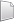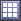# HS-4200: Material Calibration Using System Identification

Learn a method for characterizing parameters of a RADIOSS material law used for modeling elasto-plastic material.

Before you begin, copy the model files used in this tutorial from <hst.zip>/HS-4200/ to your working directory.

The characterization of a ductile aluminum alloy is studied. A RADIOSS simulation is performed to replicate an experimental tensile test. The parameters of the material law are determined to fit the experimental results.

HS-1506: Material Calibration with a Curve Difference Integral provides an alternative method to setup this problem using a HyperMath or Python function to measure the difference between two curves.

HS-1507: Material Calibration with Area Tool in Data Source provides an alternative method to set up this problem using the Area tool.

## Model Definition

A quarter of a standard tensile test specimen is modeled using symmetry conditions. A traction is applied to a specimen via an imposed velocity at the left-end.

The units are: mm, ms, g, N, MPa.
The material to be characterized is a 6063 T7 Aluminum. It has an isotropic elasto-plastic behavior which can be reproduced by a Johnson-Cook model without damage (RADIOSS Block Law2), defined as:
In this tutorial, the parameters a, b, n, σmax (maximum stress), and the Young modulus are defined as input variables. The stress-strain curve obtained by the experimental test is shown in Figure 3.
For the simulation results, engineering strains will be obtained by dividing the displacement of node 1 by the reference length (75 mm), and engineering stresses will be obtained by dividing the force in section 1 by its initial surface (10.2 mm2).

## Create Base Input Template

In this step, create the base input template in HyperStudy or use the base input template in the study directory.

1. Start HyperStudy.
2. From the menu bar, click Tools > Editor.
The Editor opens.
3. In the File field, navigate to your working directory and open the file TENSILE_TEST_0000.rad.
Note: RADIOSS uses fixed fields of 20 characters for properties.
4. In the Find area, enter /MAT/PLAS_JOHNS/1 and click.
HyperStudy highlights /MAT/PLAS_JOHNS/1 in the TENSILE_TEST_0000.rad file.
5. Select E by starting at the beginning of row 51 and highlighting the first 20 fields.
Tip: To assist you in selecting 20-character fields, press Control to activate the Selector (set to 20 characters) and then click the value.
6. Right-click on the highlighted fields and select Create Parameter from the context menu.
The Parameter: varname_1 dialog opens.
7. In the Label field, enter E_Young.
8. Change the bounds.
• Lower Bound: 50000
• Nominal: 60400
• Upper Bound: 70000
9. In the Format field, enter %20.5f.
10. Click OK.
11. Define four more variables using the information provided in Table 1.
Note: Some of the initial values are different from the values in the original file.
Table 1.
Variable Label Lower Bound Nominal Upper Bound Format
a a_PlasticityYieldStress 90 110 120 %20.5f
b b_HardeningCoeff 100 125 160 %20.5f
n n_HardeningExpo 0.1 0.2 0.3 %20.5f
sigmax Sigma_Max 250 280 290 %20.5f
12. Click OK to close the Editor.
13. In the Save Template dialog, navigate to your working directory and save the file as TENSILE_TEST_000.tpl.

## Perform the Study Setup

1. Start a new study in the following ways:
• From the menu bar, click File > New.
• On the ribbon, click.
2. In the Add Study dialog, enter a study name, select a location for the study, and click OK.
3. Go to the Define Models step.
4. Add a Parameterized File model.
1. From the Directory, drag-and-drop the TENSILE_TEST_0000.tpl file into the work area.
2. In the Solver input file column, enter TENSILE_TEST_0000.rad.
This is the name of the solver input file HyperStudy writes during the evaluation.
4. Verify that the Solver input arguments field reads \$(file).
This argument runs the Starter, and the Engine of RADIOSS for the crash analysis. It also prevents the creation of the .h3d result file from animation files. X is the number of CPUs to use for the simulation.
5. Define a model dependency
1. Click Model Resources.
The Model Resource dialog opens.
2. Select Model 1 (m_1).
3. Click Resource Assistant > Add File.
4. In the Select File dialog, navigate to your working directory and open the TENSILE_TEST_0001.rad file.
5. Set Operation to Copy.
6. Click Close.
6. Click Import Variables.
Five input variables are imported from the TENSILE_TEST_0000.tpl resource file.
7. Go to the Define Input Variables step.
8. Review the input variable's lower and upper bound ranges.

## Perform Nominal Run

1. Go to the Test Models step.
2. Click Run Definition.
An approaches/setup_1-def/ directory is created inside the study directory. The approaches/setup_1-def/run__00001/m_1 directory contains the input file, which is the result of the nominal run.

## Create and Evaluate Output Responses

In this step, you will compare RADIOSS stress-strain curve to the experimental data.

You will focus on three specific points in the curve for this comparison. Since damage is not modeled with this law, the comparison is not needed after the necking point.
• Difference between experimental stress and RADIOSS at Strain equal 0.02 (1)
• Difference between experimental strain and RADIOSS at Necking point (2)
• Difference between experimental stress and RADIOSS at Necking point (3)
1. Go to the Define Output Responses step.
2. Create a data source labeled Disp_sim.
1. Click the Data Sources tab.
2. From the Directory, drag-and-drop the TENSILE_TEST01 file, located in approaches/setup_1-def/run__00001/m_1, into the work area.
The Data Source Builder opens.
3. For Tool, select File Source.
4. Define the following options:
• Type: None/Node 1
• Request: 4 Node 1
• Component: DX-X Displacement
5. Click OK.
6. In the work area, change the label for the data source to Disp_sim.
3. Repeat step 2 to create a second data source labeled Force_sim, making the following changes during the process:
1. Set Type to Section/SECTION_2.
2. Set Request to 2 section 1.
3. Set Component to FT-Resultant Tangent Force.
4. Create three output responses.
1. Click the Define Output Responses step.
2. Click Add Output Response three times
3. In the work area, Label column, change the labels for the three output responses to Radioss_Strain_0_2, Radioss_Stress_Necking, and Radioss_Strain_Necking.
5. Define the Radioss_Strain_0_2 output response.
1. In the Expression column of the output response Radioss_Strain_0_2, click (...).
The Expression Builder opens.
2. Click the Function tab.
3. From the list of functions, select lininterp.
4. Click Insert Varname.
The function lininterp(,,) appears in the Evaluate Expression field.
5. In the Evaluate Expression field, enter (ds_1/75,ds_2/10.2,0.02) in the lininterp function.
This expression computes the Stress with respect to the Strain, at Strain equals 0.02.
6. Click OK.
6. Define the Radioss_Stress_Necking output response.
1. In the Expression column of the output response Radioss_Stress_Necking, click (...).
The Expression Builder opens.
2. Click the Data Sources tab.
3. From the list of data sources, select Force_sim.
4. From the Insert Varname drop-down, select Maximum.
5. Click Insert Varname.
The function max(ds_2) appears in the Evaluate Expression field.
6. In the Evaluate Expression field, edit the function so that it reads max(ds_2)/10.2.
This is the maximum of the force (ds_2), which is trimmed between the min strain and the strain at the max value of Force, divided by 10.2 (surface area) to obtain the stress.
Note: In some similar curve fitting problems it may be required to trim the data after the ultimate load, the expression to do this is: max(ds_2[subrange(ds_1,min(m_1_ds_1),ds_1[indexofmax(ds_2)])])/10.2.
7. Click OK.
7. Define the Radioss_Strain_Necking output response.
1. In the Expression column of the output response Radioss_Strain_Necking, click (...).
The Expression Builder opens.
2. In the Evaluate Expression field, enter ds_1[indexofmax(ds_2)]/75.
This is the displacement (ds_1) at the max value of the force value, divided by 75 (reference length) to obtain strain.
Note: In some similar curve fitting problems it may be required to trim the data after the ultimate load, the expression to do this is: ds_1[maxindex(subrange(ds_1,min(ds_1),ds_1[indexofmax(ds_2)]))]/75.
3. Click OK.
8. Click Evaluate to extract the response values.

## Run Optimization

1. In the Explorer, right-click and select Add from the context menu.
2. In the Add dialog, select Optimization and click OK.
2. Go to the Optimization 1 > Definition > Define Output Responses step.
3. Click the Objectives/Constraints - Goals tab.
2. In the Apply On column, select Radioss_Strain_0_2.
3. In the Type column, select More.
4. In column 1, select System Identification.
5. In column 2, enter 141.00000.
1. Specify the following for Radioss_Stress_Necking:
• Type: System Identification
• Target Value: 148.00000
2. Specify the following for Radioss_Strain_Necking:
• Type: System Identification
• Target Value: 0.0800000
6. Go to the Optimization > Specifications step.
7. In the work area, set the Mode to Adaptive Response Surface Method (ARSM).
Note: Only the methods that are valid for the problem formulation are enabled.
8. Click Apply.
9. Go to the Optimization > Evaluate step.
10. Click Evaluate Tasks to launch the optimization.
11. Review iteration history.
1. Click the Iteration Plot tab.
2. Activateto see the each channel in its own plot.
3. Using the Channel selector, select the three objectives and Objective Function.
The first three selections are the actual values used in the system identification optimization problem. Observe their objective history to see that their values indeed approach their respective target values. The final plot is the scalar objective which is used in the system identification problem; a normalized sum of the squares difference between the actual and target objective values. Note that the value of this combined function has been reduced through the optimization.